Miscellaneous

Chapter 6 Class 12 Application of Derivatives
Serial order wise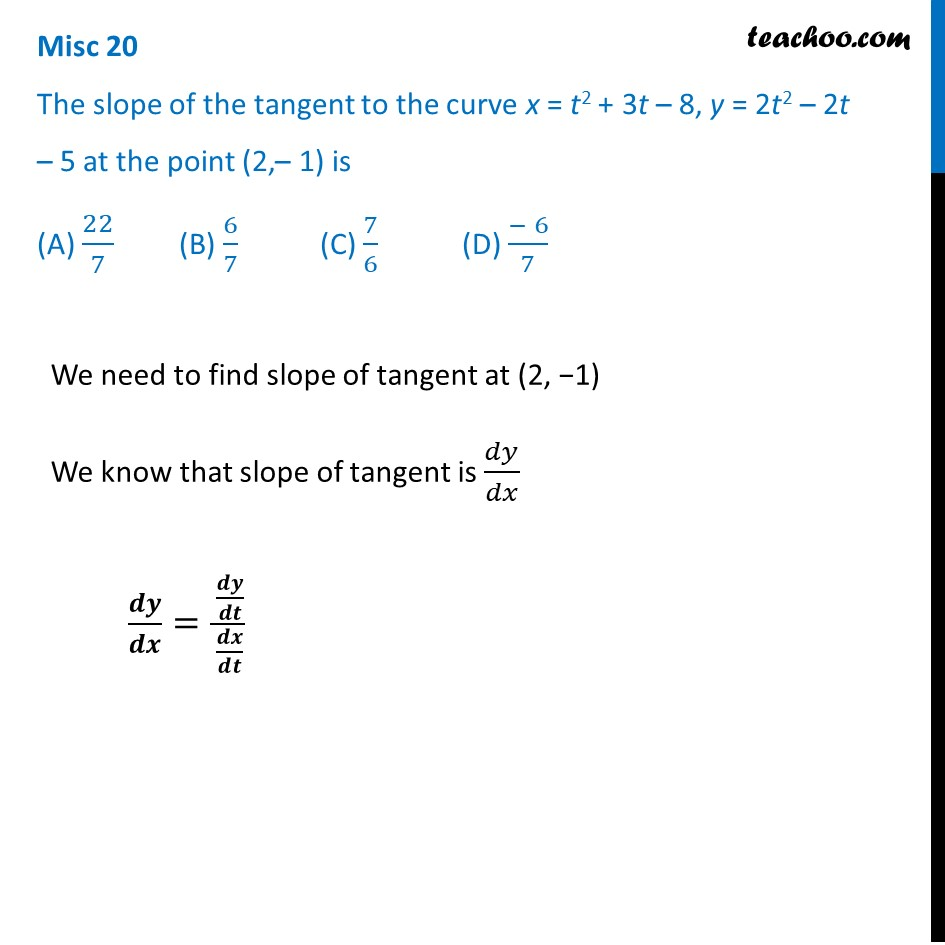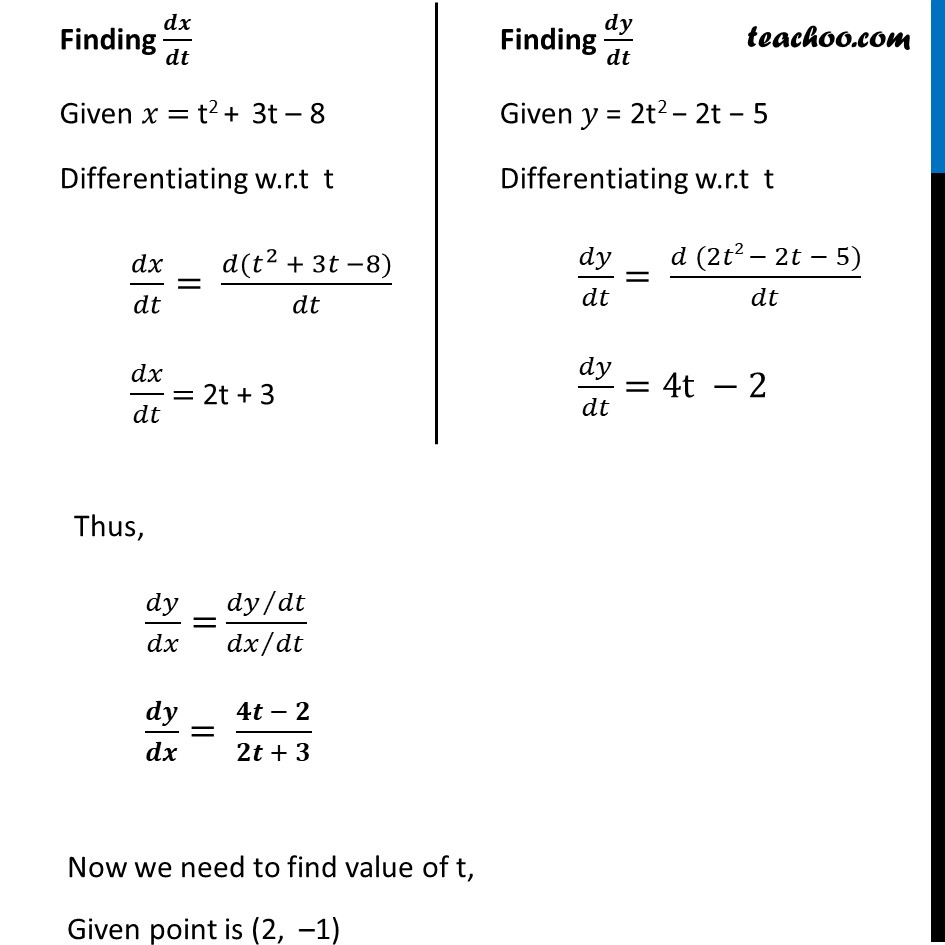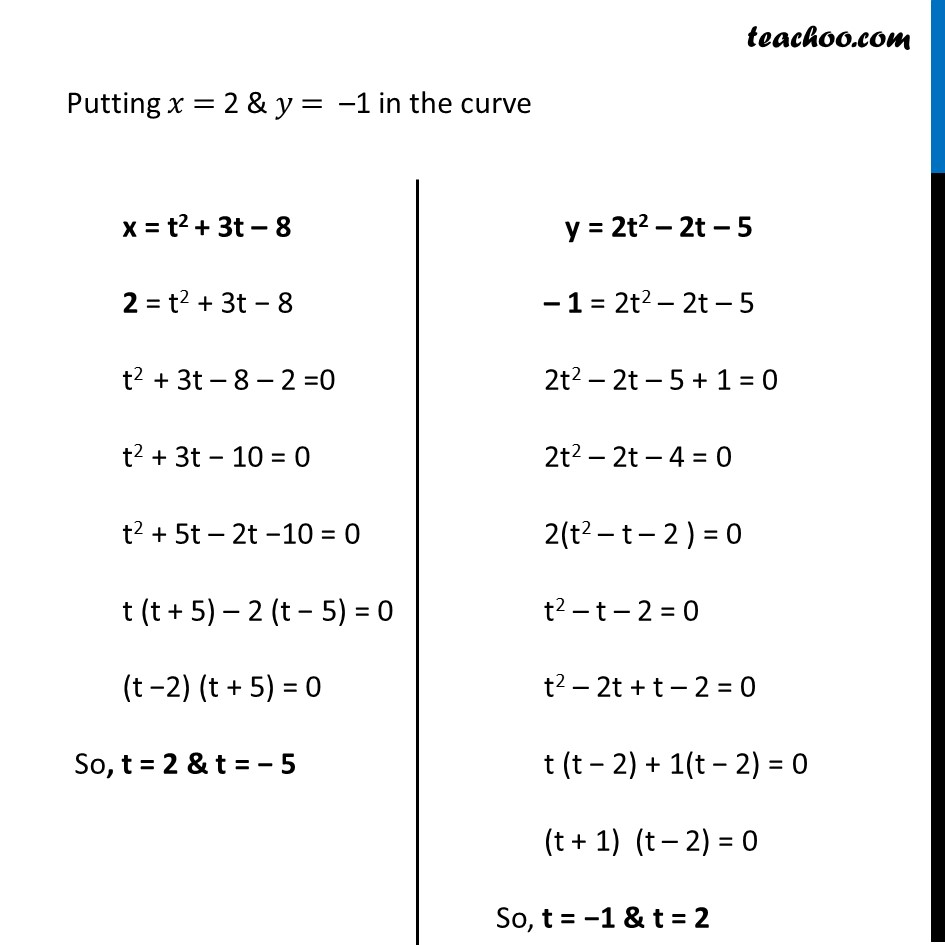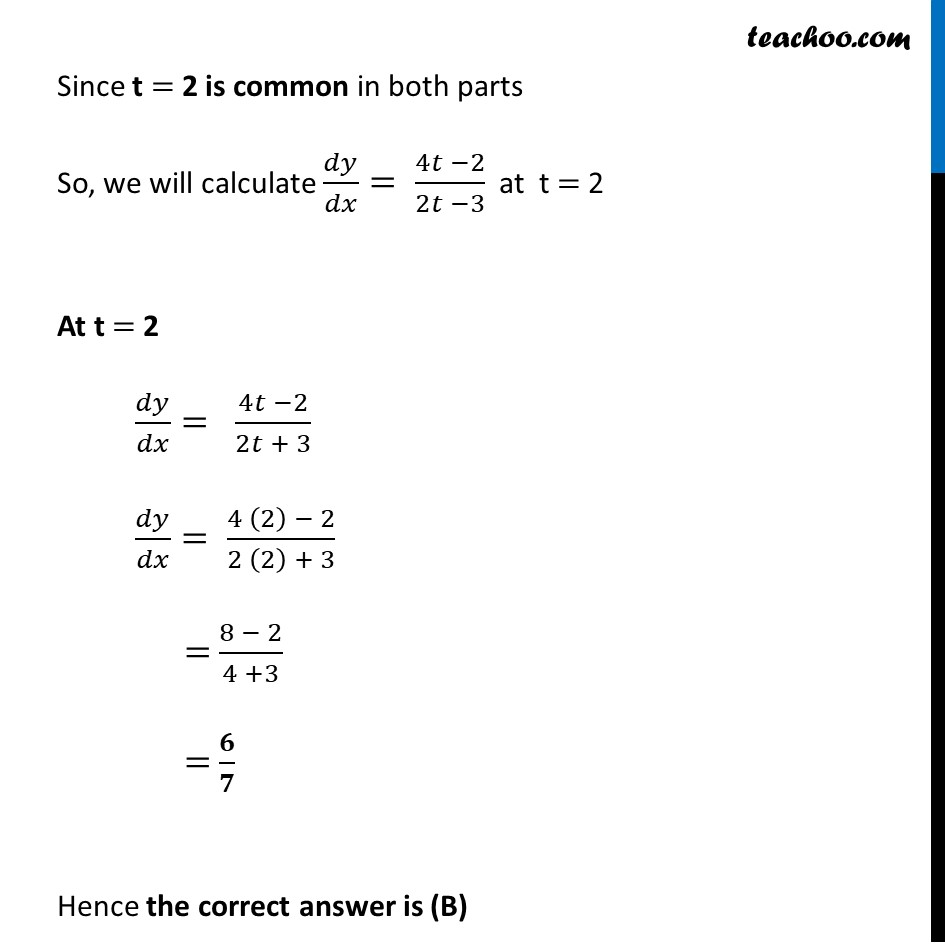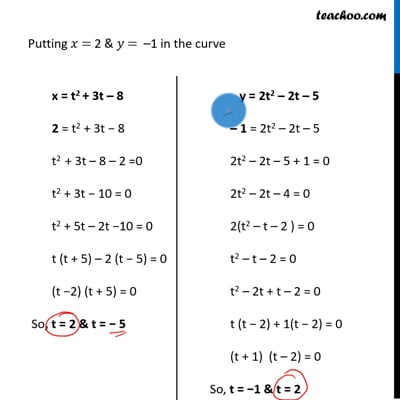This video is only available for Teachoo black users

Solve all your doubts with Teachoo Black (new monthly pack available now!)

### Transcript

Misc 20 The slope of the tangent to the curve x = t2 + 3t – 8, y = 2t2 – 2t – 5 at the point (2,– 1) is (A) 22/7 (B) 6/7 (C) 7/6 (D) (− 6)/7We need to find slope of tangent at (2, −1) We know that slope of tangent is 𝑑𝑦/𝑑𝑥 𝒅𝒚/𝒅𝒙= (𝒅𝒚/𝒅𝒕)/(𝒅𝒙/𝒅𝒕) Finding 𝒅𝒙/𝒅𝒕 Given 𝑥 = t2 + 3t – 8 Differentiating w.r.t t 𝑑𝑥/𝑑𝑡= (𝑑(𝑡^2 + 3𝑡 −8))/𝑑𝑡 𝑑𝑥/𝑑𝑡 = 2t + 3 Finding 𝒅𝒚/𝒅𝒕 Given 𝑦 = 2t2 − 2t − 5 Differentiating w.r.t t 𝑑𝑦/𝑑𝑡= (𝑑 (2𝑡2 − 2𝑡 − 5))/𝑑𝑡 𝑑𝑦/𝑑𝑡=4t −2 Thus, 𝑑𝑦/𝑑𝑥= (𝑑𝑦∕𝑑𝑡)/(𝑑𝑥∕𝑑𝑡) 𝒅𝒚/𝒅𝒙= (𝟒𝒕 − 𝟐)/(𝟐𝒕 + 𝟑) Now we need to find value of t, Given point is (2, –1) Putting 𝑥 = 2 & 𝑦 = –1 in the curve x = t2 + 3t – 8 2 = t2 + 3t − 8 t2 + 3t – 8 – 2 =0 t2 + 3t − 10 = 0 t2 + 5t – 2t −10 = 0 t (t + 5) – 2 (t − 5) = 0 (t −2) (t + 5) = 0 So, t = 2 & t = − 5 y = 2t2 – 2t – 5 – 1 = 2t2 – 2t – 5 2t2 – 2t – 5 + 1 = 0 2t2 – 2t – 4 = 0 2(t2 – t – 2 ) = 0 t2 – t – 2 = 0 t2 – 2t + t – 2 = 0 t (t − 2) + 1(t − 2) = 0 (t + 1) (t – 2) = 0 So, t = −1 & t = 2 Since t = 2 is common in both parts So, we will calculate 𝑑𝑦/𝑑𝑥= (4𝑡 −2)/(2𝑡 −3) at t = 2 At t = 2 𝑑𝑦/𝑑𝑥= (4𝑡 −2)/(2𝑡 + 3) 𝑑𝑦/𝑑𝑥= (4 (2) − 2)/(2 (2) + 3) = (8 − 2)/(4 +3) = 𝟔/𝟕 Hence the correct answer is (B)